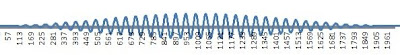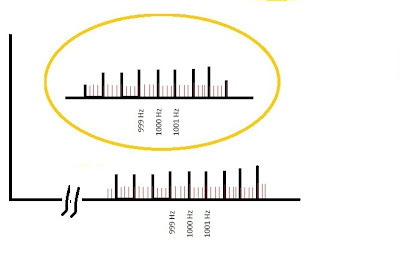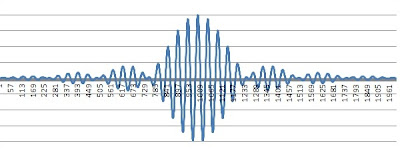## Sunday, February 20, 2011

### Harmonic Oscillator: The problem is solved

Okay. Now I've figured out something good. This is a problem that has plagued me for years and now I've solved it.

I've been talking about the equilibrium between the radiation field and the molecular oscillator. We said that the oscillator gets its energy from collisions with other molecules and pumps energy into the radiation field. Of course that's only half the story. The radiation field also pumps energy into the molecular oscillator. The problem is to calculate the equilibrium point, and now I've figured out how to do it.

Why is this such a difficult problem? It's difficult in every possible way. But probably the greatest difficulty of all is that the fields are random. So we have an oscillator vibrating at 1000 MHz. It woud be fine and dandy if we simply had a radiation field at 1ooo MHz with a strength of 100 milliwatts per square meter. That we could work with. But that is not what we have. No, what we have is a radiation distribution of 100 mW/m^2 .....per MegaHertz! So what do we work with? Do we consider only the radiation at exactly 1000 MHz? No, because there is zero radiation at any exact frequency! We have to sample it over a certain finite bandwidth or we get nothing. So do we consider all radiation between 999 and 1001 MHz? That gives us a total power density of 200mW/m^2? But we could just as well have considered all the radiation between 996 and 1004 MHz, giving us a total power density of 800 mW/m^2. Which one should it be??? It's all very confusing.

What I've figured out today is that...it doesn't matter! We're free to make either choice, and we get the same result. This seems crazy, but I'm going to show you why it works. The first step is to describe the power distribution. Mathematically, it's very tricky do deal with continuous distributions, but we can always approximate them by discrete sums. I've drawn a sketch of a graph showing a continuous frequency distribution in the vicinity of 1000 MHz, represented by a sum of equal sine waves spaced 1 MHz apart. (The graph says hertz, but that's a mistake. It should be labeled MHz!)By the way, there's nothing special about MegaHertzes. We could also have chosen to represent our field by taking sine waves every 250 kiloHertz. Those are the skinny little lines between the heavy black lines. This is a more accurate breakdown. If we choose the 250 kHz separation, the sine waves are only half as big, because there are more of them. I know...there are four times as many, so shouldn't they be a quarter the height? No, because power goes as the square of the amplitude. I've chosen the correct height to give the same power in either representation.

Now let's see what this power distribution looks like from the point of view of the oscillator. The oscillator is really only sensitive to power close to its frequency of oscillation. How close? That's a tough one, but let's try for starters just including power between 999 and 1001 MHz ... a bandwith of 2 MHz. There's a little circled sketch in the previous graph showing exactly how I'm calculating this - I've taken half the sine waves from the side bands, and the full wave in the middle. (If you took first-year calculus I think it's what they call Simpson's Rule.) The resulting wave looks like this:It rises and falls over a span of one microsecond. This pattern repeats itself a million times a second.
That's one way we can do it. But who said we had to restrict our bandwidth to 2 MHz? I've redone the calculation below, taking in a bandwith of 8 MHz between 996 and 1004 MHz. Again, I've used Simpson's Rule for the endpoints. Here again is a schematic of the distribution:The resulting waveform is shown below. (By the way, these pictures are for real. I actually programmed nine sine waves into an Excel spreadsheet, added them up, and plotted the graph.)It looks very different. The total time interval is still one microsecond, but the energy pulse is jammed into the middle of the frame, and the peak is much higher. In fact, the math shows you get four times the amplitude over one quarter the time interval. Is that the same total power? No, because power goes as the square of the amplitude. It's four times as much power. But that makes sense, after all...we took four times the bandwidth over a constant power density.

So depending on how we divide and truncate our power distribution, we get very different electromagnetic waveforms as applied in real time to the mechanical oscillator. It gets even more complicated if we approximate our distribution with sine waves every 250 kHz (the skinny lines in the graph). For example, taking in eight bands of power between 999 and 1001 MHz, we get a pulse train lasting one microsecond out of four, and twice the height calculated in our first example. Twice the power for one quarter the time interval? That's the same net energy .... that's a good sign at least. But again it's a very different looking wave.
But here's what I just figured out .... these waves all have exactly the same effect on a mechanical oscillator! It doesn't matter which approximation or how much bandwidth we choose. We're going to find it gives the same average boost to a random oscillator. It means we can go ahead with the equilibrium calculation. That will be the topic of my next post.